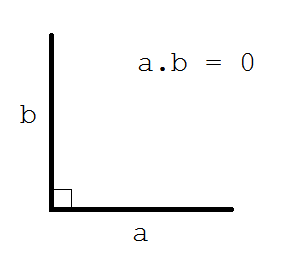# Checking for 90 degree rotation not working

Hey there devs.

I am working on a rail system which i have been making a few threads about recently. What i am trying to get it to do right now, is detect irregular or sharp curves and deny the player from placing them.

The problem is that i cant seem to get it to work. I have tried multiple ways to do this. Right now i am trying to check if the turn that the track makes is more than 90 degrees, and if so, deny the player from placing it. But when it becomes very sharp, it believes that it is less than a 90 degree angle?

i was previously iterating through the track in increments, and checking if any point in the rail was turning too quickly, but that wasn’t working either.

Here is an example of how it believes that sharp turns are less than 90 degrees:

Here is my current relevant code:

``````local fa, ba = b:Get(0+(0.1/lengt)), b:Get(0)
local fb, bb = b:Get(1), b:Get(1-(0.1/lengt))
local ac, bc = CFrame.new((fa+ba)/2, fa), CFrame.new((fb+bb)/2, fb)
local fac = ac.LookVector
local vec = (bc.Position - ac.Position).unit
angl = math.acos(math.max(-1, math.min(1, fac:Dot(vec))))*100

if angl > 90 then
toosharp = true
else
toosharp = false
end
``````

:Get() just takes a value from 0 to 1 and gets the position at that percentage of the curve.
How can i stop it from allowing that sharp curve, when it is more than 90 degrees?

Thank you for any help,

• Mezza

Still working this through, but would an alternative be to do a magnitude check between every wood part, and if it was too small then also deny them? Because from what I can tell, the wood parts get closer the greater the angle.

I could possibly do that, i didn’t think of it. I attempted it, but the way the system works, sometimes slightly curved pieces have rail pieces very close together. I could try to change it, but i think doing it by finding the difference between the rotations of the start and end of the rail would be more efficient, correct me if i’m wrong.

I will just use that for now. Thanks for the idea

1 Like

Using some linear algebra, you can use the dot product to see if two vectors are forming 90 degress (in other words perpendicular).
If two vectors are perpendicular there dot product would be equal to 0So maybe you can check if `firstrailposiition:Dot(secondrailposition) == 0`